# Laplace distribution

(diff) ← Older revision | Latest revision (diff) | Newer revision → (diff)

A continuous probability distribution with densitywhere,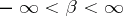, is a shift parameter andis a scale parameter. The density of the Laplace distribution is symmetric about the point, and the derivative of the density has a discontinuity at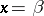. The characteristic function of the Laplace distribution with parametersandis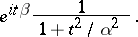The Laplace distribution has finite moments of any order. In particular, its mathematical expectation isand its variance (cf. Dispersion) is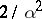.

The Laplace distribution was first introduced by P. Laplace  and is often called the "first law of Laplacefirst law of Laplace" , in contrast to the "second law of Laplacesecond law of Laplace" , as the normal distribution is sometimes called. The Laplace distribution is also called the two-sided exponential distribution, on account of the fact that the Laplace distribution coincides with the distribution of the random variable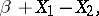where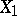and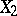are independent random variables that have the same exponential distribution with density,. The Laplace distribution with density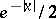and the Cauchy distribution with density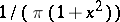are related in the following way: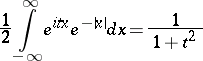and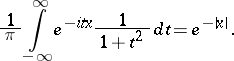How to Cite This Entry:
Laplace distribution. Encyclopedia of Mathematics. URL: http://encyclopediaofmath.org/index.php?title=Laplace_distribution&oldid=14706
This article was adapted from an original article by A.V. Prokhorov (originator), which appeared in Encyclopedia of Mathematics - ISBN 1402006098. See original article